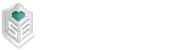## Percentages of Post Type With Up/Down Votes

1

(click on this box to dismiss)Q&A for people who build and repair bicycles, people who train cycling, or commute on bicycles

``````DECLARE @totalQuestions INT;
DECLARE @questionsWithUpvote INT;
DECLARE @questionsWithDownvote INT;

-- Get Totals
SELECT
-- Questions
@totalQuestions = COUNT(DISTINCT (CASE WHEN p.PostTypeId = 1 THEN p.Id END)),
@totalAnswers = COUNT(DISTINCT (CASE WHEN p.PostTypeId = 2 THEN p.Id END))
FROM
Posts p
WHERE p.PostTypeId IN (1, 2);

SELECT
-- Questions
@questionsWithUpvote = COUNT(DISTINCT (CASE WHEN p.PostTypeId = 1 AND v.VoteTypeId = 2 THEN p.Id END)),
@questionsWithDownvote = COUNT(DISTINCT (CASE WHEN p.PostTypeId = 1 AND v.VoteTypeId = 3 THEN p.Id END)),
@answersWithUpvote = COUNT(DISTINCT (CASE WHEN p.PostTypeId = 2 AND v.VoteTypeId = 2 THEN p.Id END)),
@answersWithDownvote = COUNT(DISTINCT (CASE WHEN p.PostTypeId = 2 AND v.VoteTypeId = 3 THEN p.Id END))
FROM
Posts p
JOIN Votes v ON p.Id = v.PostId
WHERE p.PostTypeId IN (1, 2) AND
v.VoteTypeId IN (2, 3);

-- Naive approach
SELECT @questionsWithNoVotes = @totalQuestions - @questionsWithUpvote - @questionsWithDownvote;

-- Reformat into more readable results
SELECT
ColumnDescription AS [Category],
FORMAT(Amount, '#,0') AS [Number of Posts],
FORMAT(PercentOfTotal, 'P3') AS [Percentage of Total]
FROM
(
VALUES
-- Questions
(1, 'Upvoted Questions', @questionsWithUpvote, CAST(@questionsWithUpvote AS FLOAT) / CAST(@totalQuestions AS FLOAT)),
(1, 'Downvoted Questions', @questionsWithDownvote, CAST(@questionsWithDownvote AS FLOAT) / CAST(@totalQuestions AS FLOAT)),
(1, 'Total Questions', @totalQuestions, CAST(@totalQuestions AS FLOAT) / CAST(@totalQuestions AS FLOAT)),Hold tight while we fetch your results